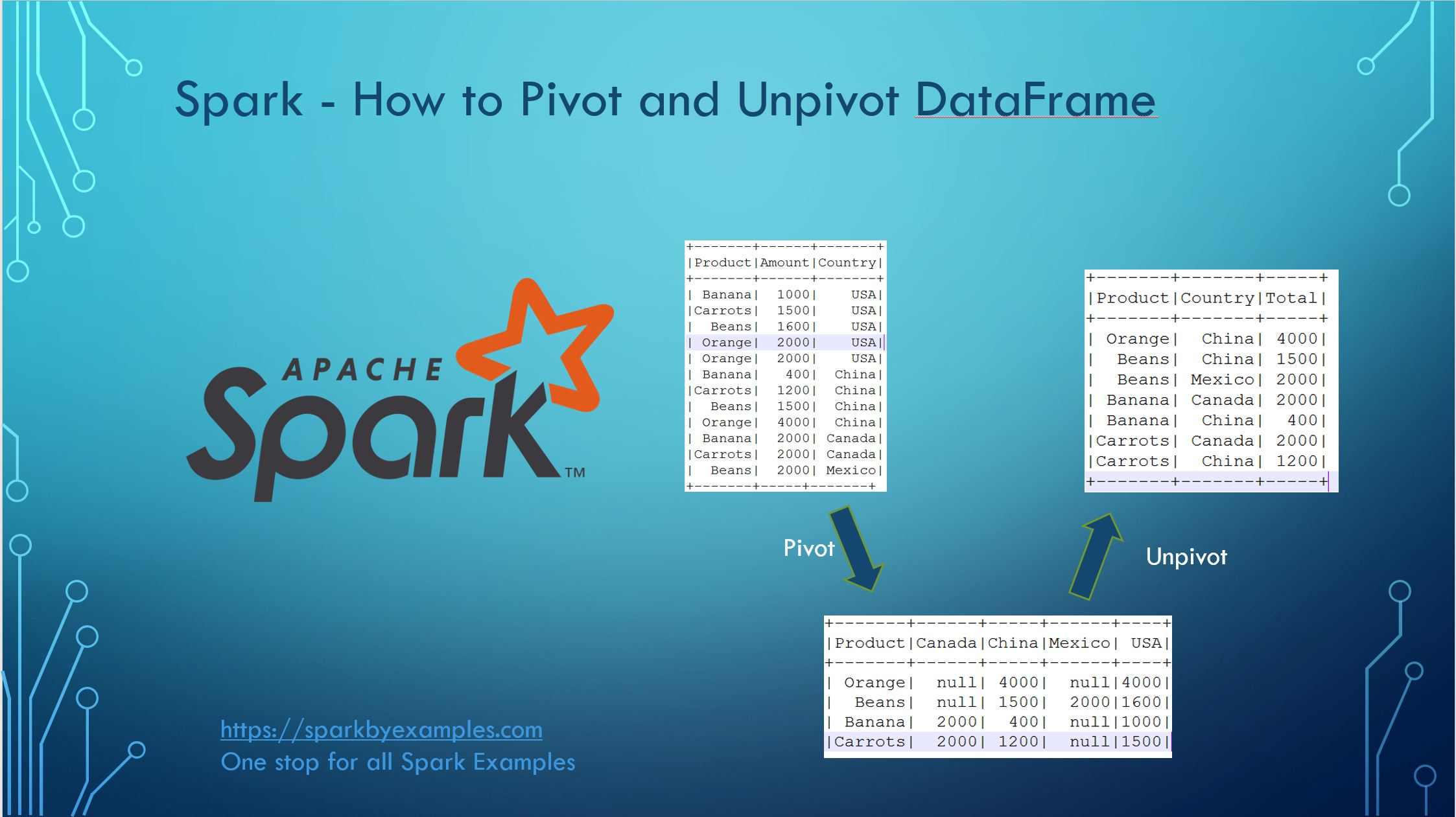# How to Pivot and Unpivot a Spark DataFrame

Spark pivot() function is used to pivot/rotate the data from one DataFrame/Dataset column into multiple columns (transform row to column) and unpivot is used to transform it back (transform columns to rows).

In this article, I will explain how to use pivot() SQL function to transpose one or multiple rows into columns.

Pivot() is an aggregation where one of the grouping columns values transposed into individual columns with distinct data.

Let’s create a DataFrame to work with.

``````val data = Seq(("Banana",1000,"USA"), ("Carrots",1500,"USA"), ("Beans",1600,"USA"),
("Orange",2000,"USA"),("Orange",2000,"USA"),("Banana",400,"China"),
("Carrots",1200,"China"),("Beans",1500,"China"),("Orange",4000,"China"),

import spark.sqlContext.implicits._
val df = data.toDF("Product","Amount","Country")
df.show()
``````

DataFrame ‘df’ consists of 3 columns Product, Amount and Country as shown below.

``````
+-------+------+-------+
|Product|Amount|Country|
+-------+------+-------+
| Banana|  1000|    USA|
|Carrots|  1500|    USA|
|  Beans|  1600|    USA|
| Orange|  2000|    USA|
| Orange|  2000|    USA|
| Banana|   400|  China|
|Carrots|  1200|  China|
|  Beans|  1500|  China|
| Orange|  4000|  China|
|  Beans|  2000| Mexico|
+-------+-----+-------+
``````

## Pivot Spark DataFrame

Spark SQL provides `pivot()` function to rotate the data from one column into multiple columns (transpose row to column). It is an aggregation where one of the grouping columns values transposed into individual columns with distinct data. From the above DataFrame, to get the total amount exported to each country of each product will do group by Product, pivot by Country, and the sum of Amount.

``````val pivotDF = df.groupBy("Product").pivot("Country").sum("Amount")
pivotDF.show()``````

This will transpose the countries from DataFrame rows into columns and produces below output. Where ever data is not present, it represents as null by default.

``````
+-------+------+-----+------+----+
+-------+------+-----+------+----+
| Orange|  null| 4000|  null|4000|
|  Beans|  null| 1500|  2000|1600|
| Banana|  2000|  400|  null|1000|
|Carrots|  2000| 1200|  null|1500|
``````

## Pivot Performance improvement in Spark 2.0

Spark 2.0 on-wards performance has been improved on Pivot, however, if you are using lower version; note that pivot is a very expensive operation hence, it is recommended to provide column data (if known) as an argument to function as shown below.

``````
val pivotDF = df.groupBy("Product").pivot("Country", countries).sum("Amount")
pivotDF.show()
``````

Another approach is to do two-phase aggregation. Spark 2.0 uses this implementation in order to improve the performance Spark-13749

``````
val pivotDF = df.groupBy("Product","Country")
.sum("Amount")
.groupBy("Product")
.pivot("Country")
.sum("sum(Amount)")
pivotDF.show()
``````

Above two examples returns the same output but with better performance.

## Unpivot Spark DataFrame

Unpivot is a reverse operation, we can achieve by rotating column values into rows values. Spark SQL doesn’t have unpivot function hence will use the `stack()` function. Below code converts column countries to row.

``````
//unpivot
val unPivotDF = pivotDF.select(\$"Product",
.where("Total is not null")
unPivotDF.show()``````

It converts pivoted column “country” to rows.

``````
+-------+-------+-----+
|Product|Country|Total|
+-------+-------+-----+
| Orange|  China| 4000|
|  Beans|  China| 1500|
|  Beans| Mexico| 2000|
| Banana|  China|  400|
|Carrots|  China| 1200|
+-------+-------+-----+
``````

## Transpose or Pivot without aggregation

Can we do Spark DataFrame transpose or pivot without aggregation?

off course you can, but unfortunately, you can’t achieve using Pivot function. However, pivoting or transposing DataFrame structure without aggregation from rows to columns and columns to rows can be easily done using Spark and Scala hack. please refer to this example.

#### Conclusion:

We have seen how to Pivot DataFrame (transpose row to column) with scala example and Unpivot it back using Spark SQL functions. And also have seen how Spark 2.0 changes improves performance by doing two-phase aggregation.